VLOOKUP and HLOOKUP in Excel: Functions, Examples, and How to Use - Compute Expert

# VLOOKUP and HLOOKUP in Excel: Functions, Examples, and How to Use

Home >> Excel Tutorials from Compute Expert >> Excel Formulas List >> VLOOKUP and HLOOKUP in Excel: Functions, Examples, and How to Use

In this tutorial, you will learn about VLOOKUP and HLOOKUP in excel completely.

When we work in excel, we often need to find data from reference tables. We can use VLOOKUP or HLOOKUP to help us do that, depending on the reference table form we refer to.

Want to learn much more about the VLOOKUP and HLOOKUP usage and characteristics in excel? Read this tutorial until its last part!

Disclaimer: This post may contain affiliate links from which we earn commission from qualifying purchases/actions at no additional cost for you. Learn more

## What are VLOOKUP and HLOOKUP Formulas?

We can define VLOOKUP and HLOOKUP as the formulas that can help us to find data in a cell range.

If we define them separately, then VLOOKUP is the formula we use to find data in a cell range vertically (looking the data by columns). Meanwhile, HLOOKUP is the formula we use to find data in a cell range horizontally (looking the data by rows).

## Differences Between VLOOKUP and HLOOKUP

Although both are data lookup formulas, there are some basic VLOOKUP and HLOOKUP differences we should know. Here is a table that tries to summarize the main difference points between them.

VLOOKUPHLOOKUP
Stands for “Vertical Lookup”Stands for “Horizontal Lookup”
Lookup for data in a reference table based on columnsLookup for data in a reference table based on rows
Find its lookup reference value in the first column of its reference tableFind its lookup reference value in the first row of its reference table

After you learned their differences, don’t choose between those two formulas wrongly!

## VLOOKUP and HLOOKUP Function in Excel

We can use VLOOKUP and HLOOKUP to get the data we want from a cell range based on our lookup reference.

## The Result You Get from VLOOKUP and HLOOKUP

VLOOKUP and HLOOKUP will give you the data you search for from a cell range you specify.

## Excel Version from Which We Can Start Using VLOOKUP and HLOOKUP

We can start using VLOOKUP and HLOOKUP since excel 2003.

## The Way to Write Them and Their Inputs

Here are the general writing forms of VLOOKUP and HLOOKUP in excel.

VLOOKUP

= VLOOKUP ( lookup_reference , reference_cell_range , result_column_index , lookup_mode )

HLOOKUP

= HLOOKUP ( lookup_reference , reference_cell_range , result_row_index , lookup_mode )

The inputs in the VLOOKUP and HLOOKUP formula writing are quite similar. However, they have differences you should pay attention to, mostly related to the horizontal and vertical nature of VLOOKUP and HLOOKUP.

The following will explain the inputs of both formulas and their differences when you input them for VLOOKUP or HLOOKUP.
• lookup_reference = the value that will become the reference for VLOOKUP and HLOOKUP when they lookup for your data. VLOOKUP will search its lookup reference in the first column of its cell range. Meanwhile, HLOOKUP will search it in the first row of its cell range
• reference_cell_range = the reference table cell range where VLOOKUP and HLOOKUP will do their data lookup process
• result_column_index (VLOOKUP)/result_row_index (HLOOKUP) = the index of the column (VLOOKUP)/row (HLOOKUP) in your reference table where you want to get your data. VLOOKUP will look in which row it finds your lookup reference to get data at that same row in this column. HLOOKUP will look at which column it finds your lookup reference to get data at that same column in this row
• lookup_mode = an optional input. Determines whether you want an approximate or exact match for your lookup reference. Input TRUE for approximate and FALSE for exact. If you don’t input anything here, then excel will assume that you input TRUE

## Approximate vs Exact Match in VLOOKUP and HLOOKUP

Let’s discuss more the approximate and exact match nature in VLOOKUP and HLOOKUP as it often confuses people.

When you input FALSE or exact match, you say that you want an exact match only for your lookup reference. That means when there isn’t an exact match that your VLOOKUP/HLOOKUP finds, it will return an error.

Meanwhile, when you input TRUE or approximate match, it becomes okay to get an approximation of your lookup reference instead. When your VLOOKUP/HLOOKUP doesn’t find an exact match for your lookup reference, it will find its smaller nearest value.

Using TRUE for your VLOOKUP/HLOOKUP input comes with a caveat, however. You should sort your first column/row (depending on whether you use VLOOKUP/HLOOKUP) in ascending order so the TRUE can function well. If you don’t, then your VLOOKUP/HLOOKUP can produce wrong or even error results.

Remember that the default input here is TRUE. Thus, if you want to use an exact match lookup mode, then don’t forget to input FALSE.

## VLOOKUP and HLOOKUP Example

The following will give and explain the example of HLOOKUP and VLOOKUP implementation and their results.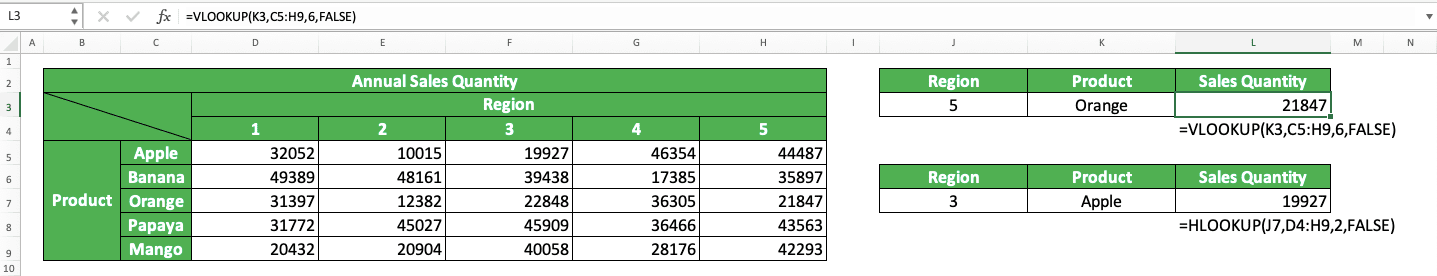In the example, you can see how we write VLOOKUP and HLOOKUP in excel and the result we get from them. The writings for both are quite similar although you have to take different perspectives when referring to the reference table.

Both VLOOKUP and HLOOKUP will try to find their lookup reference and see its row/column position. Then, they will get your data from the column/row you specify through the intersection with that row/column position. They can find an approximate match if it doesn’t find the exact one if you input TRUE as the lookup mode.

Sometimes, there are also cases where there is more than one exact match in your first column/row. If that happens, then VLOOKUP will make the more top positioned match as the data position reference in the result column. Whereas, HLOOKUP will make the most left match as the data position reference in the result row.

## Steps to Write VLOOKUP and HLOOKUP

The following will explain in step-by-step how to write VLOOKUP and HLOOKUP in excel. Learning the writing of HLOOKUP and VLOOKUP can be quite difficult if you just know them. Therefore, we provide the VLOOKUP and HLOOKUP writing example screenshots in each step to help you understand.

1. Type an equal sign ( = ) in the cell where you want to put your VLOOKUP/HLOOKUP result2. Type VLOOKUP/HLOOKUP (can be with large and small letters), depending on which formula you want to use, and an open bracket sign after =3. Input the lookup reference value or the cell coordinate where the value is. Then, type a comma sign ( , )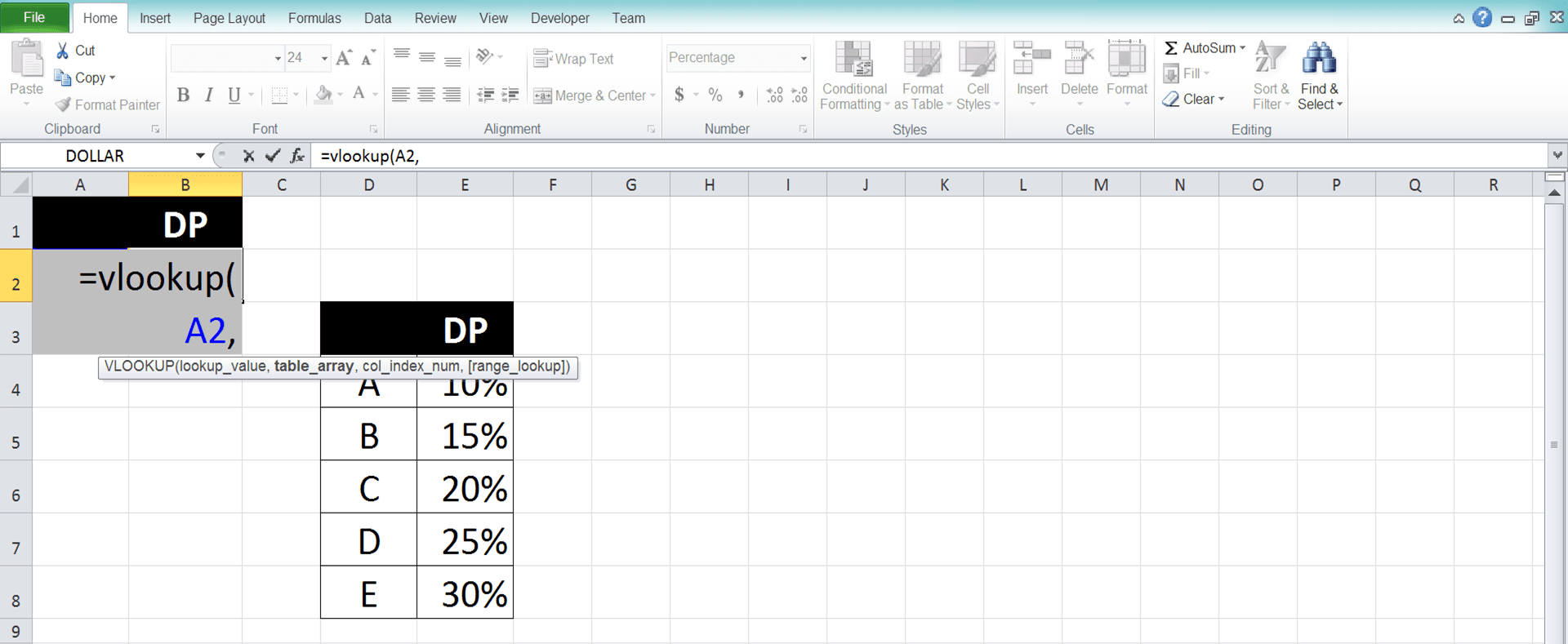4. Input the cell range where the reference table to find your data is then type a comma sign. Later, VLOOKUP/HLOOKUP will search the lookup value we inputted earlier in the first column (VLOOKUP)/row (HLOOKUP) of this cell range5. Input the nth order of the column (VLOOKUP)/row (HLOOKUP) where you want to find your lookup result in the reference table. VLOOKUP will get its result from the row in which it finds your lookup reference combined with the column. Whereas, HLOOKUP will get its result from the column in which it finds your lookup reference combined with the row.

If there is more than one match for the lookup value, it will prioritize the top one (VLOOKUP)/the most left one (HLOOKUP)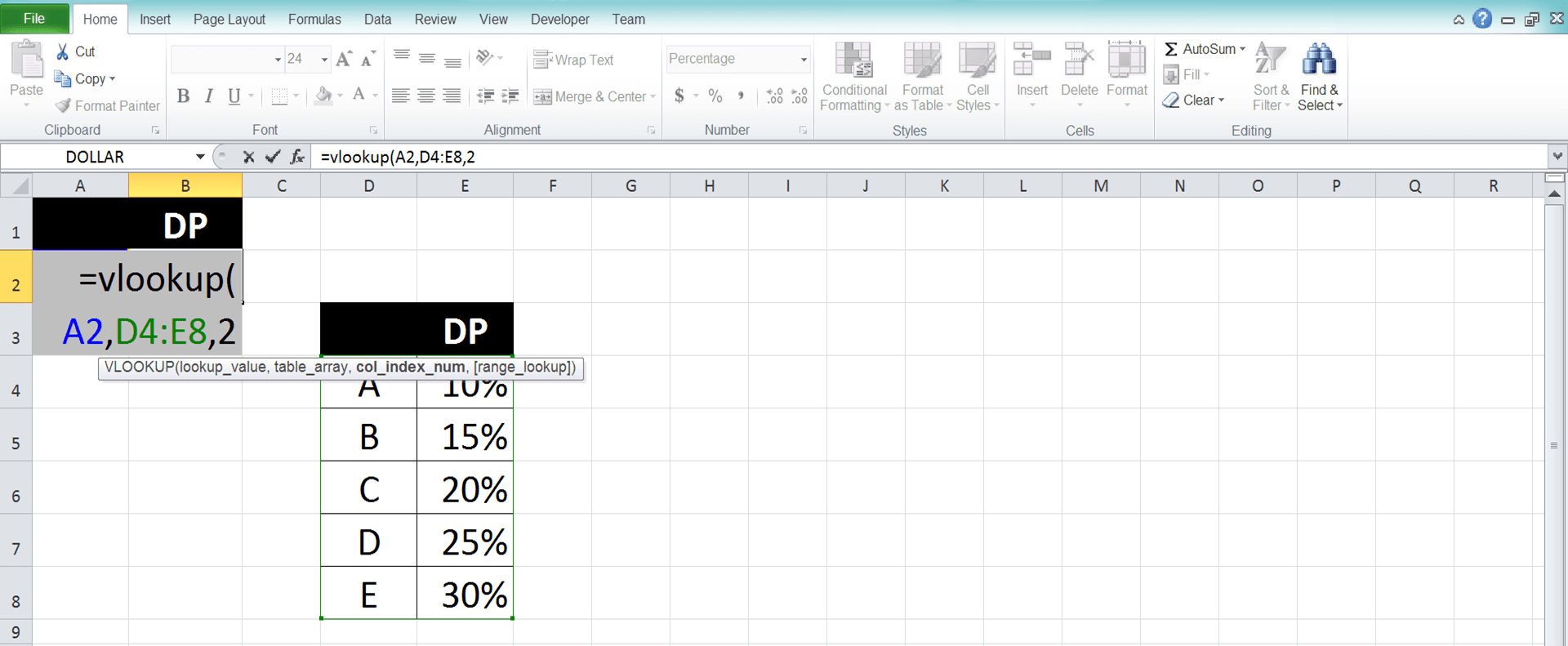6. Optional: To determine whether VLOOKUP/HLOOKUP should find an exact/approximate match to your lookup reference value, do this. Input a comma sign and type TRUE/FALSE (can be with large and small letters). TRUE for approximate and FALSE for exact.

If there is no input from you in this part, then VLOOKUP/HLOOKUP will assume your input as TRUE7. Type a close bracket sign8. Press Enter
9. Done!## Combining VLOOKUP and HLOOKUP Together

Need to find data from your reference table and you still don’t know its row and column position? You can combine VLOOKUP and HLOOKUP to help you.

You need to create a helper column/row which contains the indexes of the columns/rows in your reference table first. If you create a helper column, then write your VLOOKUP inside your HLOOKUP for the combination. If you create a helper row, then write your HLOOKUP inside your VLOOKUP instead.

Here are the general writing forms of the VLOOKUP and HLOOKUP combination. The first one is VLOOKUP inside HLOOKUP and the second one is HLOOKUP inside VLOOKUP.

VLOOKUP inside HLOOKUP

= HLOOKUP ( column_lookup_reference , reference_cell_range , VLOOKUP ( row_lookup_reference , lookup_reference_and_helper_cell_range , helper_row_index , lookup_mode ) , lookup_mode )

HLOOKUP inside VLOOKUP

= VLOOKUP ( row_lookup_reference , reference_cell_range , HLOOKUP ( column_lookup_reference , lookup_reference_and_helper_cell_range , helper_column_index , lookup_mode ) , lookup_mode )

In those writing forms, we must input the correct lookup reference values to our VLOOKUP and HLOOKUP. Input the row lookup reference value to VLOOKUP and input the column lookup reference value to HLOOKUP.

In the VLOOKUP/HLOOKUP we put inside, we input the cell range where our lookup reference and helper column/row are. For the result column/row index, we input the index where our helper column/row is. If we create the helper column/row just after our lookup reference column/row, then input 2 here.

To better understand this VLOOKUP and HLOOKUP combination concept, take a look at the screenshots below.

VLOOKUP inside HLOOKUP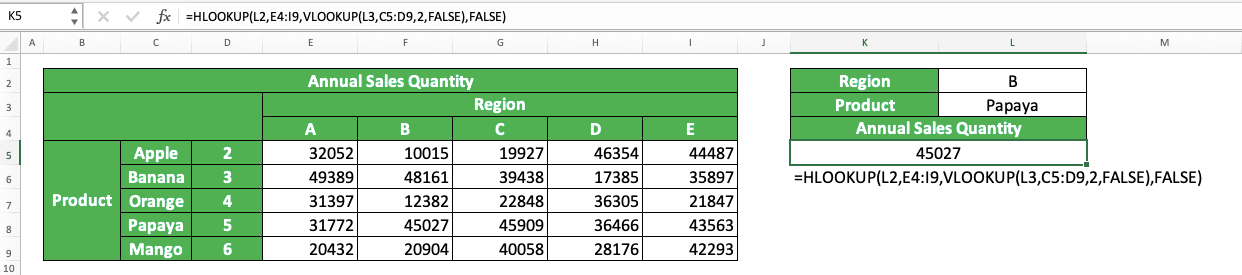HLOOKUP inside VLOOKUPIn those examples, we want to get annual sales quantity data from our reference table on the left. We want to get it according to the product name and sales region we have.

You can see from both examples that we can get the data by writing VLOOKUP inside HLOOKUP or HLOOKUP inside VLOOKUP. Both combination methods produce the same results.

If we use VLOOKUP inside HLOOKUP combination, then we should create a helper column that contains our data column indexes. If we use HLOOKUP inside VLOOKUP combination, then we should create a helper row that contains our data row indexes.

We fill the helper column/row with indexes starting 2 since index 1 is for the lookup reference column/row. As we create the helper column/row just after the lookup reference column/row, we input 2 in our VLOOKUP/HLOOKUP.

We use FALSE as the lookup mode for both our VLOOKUP and HLOOKUP since we only want exact matches. We write our VLOOKUP and HLOOKUP combination correctly like this and we will get the correct data we want!

## VLOOKUP and HLOOKUP Alternative: INDEX MATCH

If you often use VLOOKUP and HLOOKUP, then you might want to consider their alternative: INDEX MATCH. It is a powerful and flexible data lookup formula and it is the combination between two excel formulas: INDEX and MATCH.

If we use them separately, then INDEX can help you to get data based on its column and row position inputs. Meanwhile, MATCH can help you to get data column or row position in a cell range. Looking at both formulas’ functions, combining them highly makes sense to get the data we want from a reference table.

Here is the general writing form of INDEX MATCH in Excel.

= INDEX ( reference_cell_range , MATCH ( row_lookup_reference , row_reference_range , lookup_mode ) , MATCH ( column_lookup_reference , column_reference_range , lookup_mode ) )

In this writing form, we assume we need to find the row and column positions of our data. If we just need to find one of them, then we input MATCH to the one input we need to find. We adjust our INDEX cell range and row/column position inputs in that kind of situation too.

To help your consideration in using INDEX MATCH, here are its comparison points with VLOOKUP and HLOOKUP in a table.

VLOOKUP and HLOOKUPINDEX MATCH
Lookup for data in a cell range vertically or horizontally. Can lookup for data vertically and horizontally too if we create a helper column/rowLookup for data in a cell range vertically and/or horizontally without the need of a helper column/row
Look up its lookup reference value in the first column/row of its cell rangeLook up its lookup reference value in any column/row we want in its cell range
Lookup mode options are approximate (smaller nearest) and exact matchesLookup mode options are approximate (smaller nearest and larger nearest) and exact matches

And here are the implementation examples of INDEX MATCH which mirrors the VLOOKUP and HLOOKUP implementation.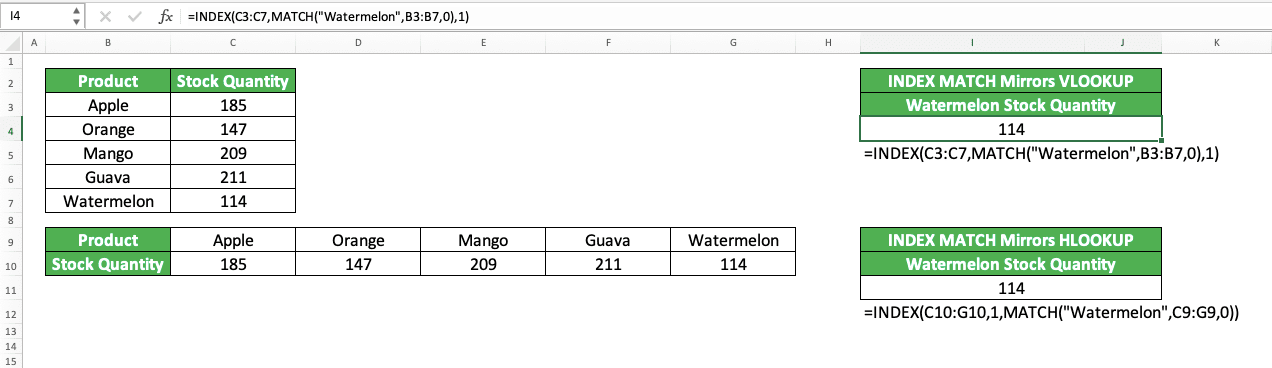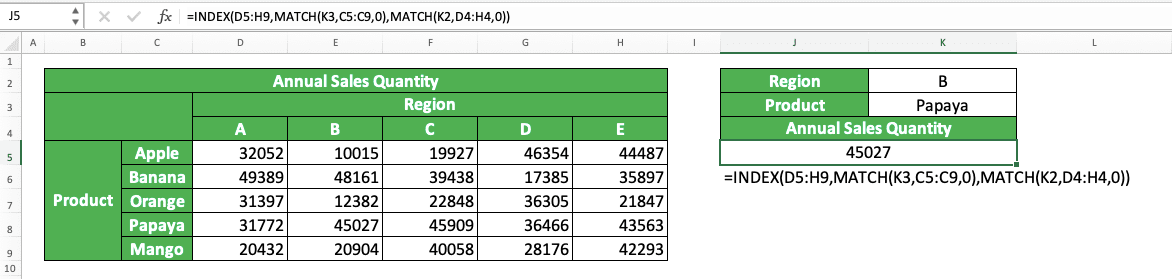In those examples, we can see clearly how INDEX MATCH can replace the VLOOKUP and HLOOKUP functions. The INDEX MATCH writing depends on what kind of data lookup process we want to do.

If we want to mirror the VLOOKUP function, then we input MATCH as the row position input of our INDEX. If we want to mirror the HLOOKUP function, then we input MATCH as the column position input of our INDEX. If we want to mirror VLOOKUP and HLOOKUP combination, then we input MATCH in both row and column positions inputs.

Use INDEX MATCH correctly and you can get yourself a powerful lookup formula. Who knows, you might not want to use VLOOKUP and HLOOKUP anymore after you get used to INDEX MATCH!

## VLOOKUP Comprehensive Tutorial You Need to Learn

Need to learn much deeper about the application of VLOOKUP in excel? Learn from this Compute Expert tutorial which discusses exclusively about VLOOKUP! We will discuss the basics of VLOOKUP and its advanced use in excel also there.

## HLOOKUP Comprehensive Tutorial You Need to Learn

Want to learn much more about HLOOKUP instead? We also have a Compute Expert tutorial that discusses exclusively about HLOOKUP! Visit the tutorial now to master everything you need to use the formula optimally!

## Exercise

After you learn how to use HLOOKUP and VLOOKUP formulas in excel, now is the time to do an exercise. You should do it so you can better understand the implementation of these formulas.

### Questions

Use HLOOKUP and/or VLOOKUP when answering the following questions!
1. How many is the production target of machine C in October? Use VLOOKUP to answer!
2. How many is the production target of machine D in June? Use HLOOKUP to answer!
3. How many shift estimations you must run to achieve the January target?

Many people get a wrong/error result from their VLOOKUP/HLOOKUP formula because of the lookup mode input. They input TRUE or don’t input anything there when they haven’t sorted their cell range’s first row/column.

Don’t forget to input FALSE if you only need an exact match for your lookup reference value. Or if you need to input TRUE, then don’t forget to sort your first column (VLOOKUP)/row (HLOOKUP). Doing that should help you get the right result from your VLOOKUP/HLOOKUP!

Related tutorials you should learn:

Get updated excel info from Compute Expert by registering your email. It's free!